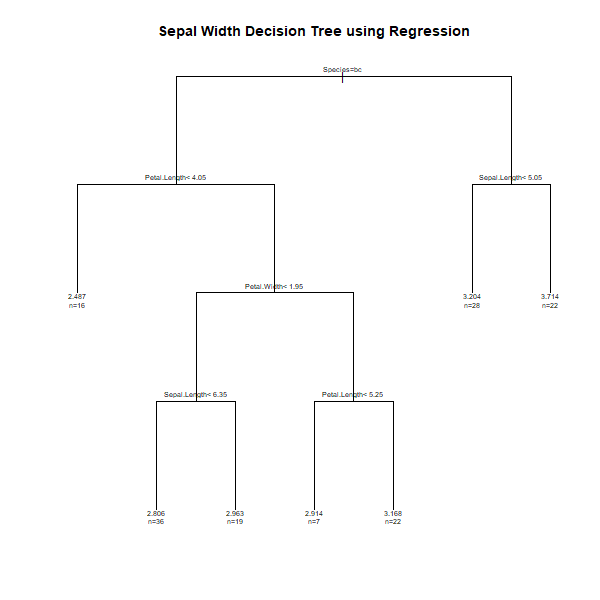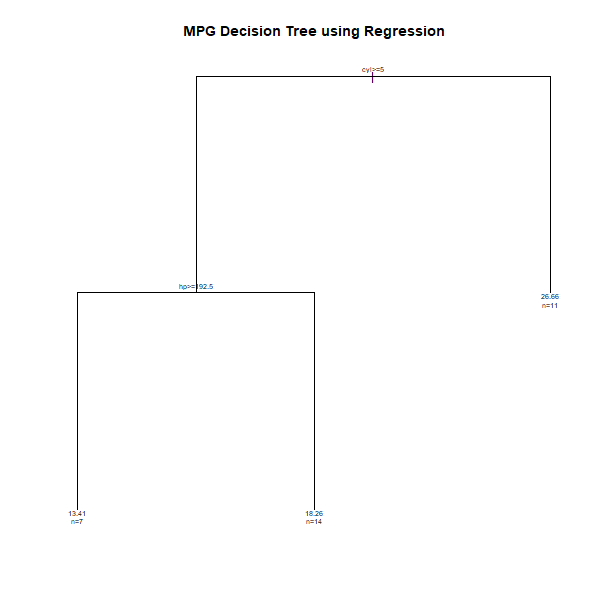GFG App
Open AppBrowser
Continue

# Decision Tree for Regression in R Programming

Decision tree is a type of algorithm in machine learning that uses decisions as the features to represent the result in the form of a tree-like structure. It is a common tool used to visually represent the decisions made by the algorithm. Decision trees use both classification and regression. Regression trees are used when the dependent variable is continuous whereas the classification tree is used when the dependent variable is categorical. For example, determining/predicting gender is an example of classification, and predicting the mileage of a car based on engine power is an example of regression. In this article, let us discuss the decision tree using regression in R programming with syntax and implementation in R programming.

#### Implementation in R

In R programming, `rpart()` function is present in `rpart` package. Using the `rpart()` function, decision trees can be built in R.

Syntax:
rpart(formula, data, method)

Parameters:
formula: indicates the formula based on which model has to be fitted
data: indicates the dataframe
method: indicates the method to create decision tree. “anova” is used for regression and “class” is used as method for classification.

Example 1:
In this example, let us predict the sepal width using the regression decision tree.

Step 1: Install the required package

 `# Install the package ` `install.packages(``"rpart"``) `

 `# Load the package ` `library(rpart) `

Step 3: Fit the model for decision tree for regression

 `# Create decision tree using regression ` `fit <``-` `rpart(Sepal.Width ~ Sepal.Length ``+`  `             ``Petal.Length ``+` `Petal.Width ``+` `Species,  ` `             ``method ``=` `"anova"``, data ``=` `iris) `

Step 4: Plot the tree

 `# Output to be present as PNG file ` `png(``file` `=` `"decTreeGFG.png"``, width ``=` `600``,  ` `                            ``height ``=` `600``) ` ` `  `# Plot ` `plot(fit, uniform ``=` `TRUE, ` `          ``main ``=` `"Sepal Width Decision  ` `                 ``Tree using Regression") ` `text(fit, use.n ``=` `TRUE, cex ``=` `.``7``) ` ` `  `# Saving the file ` `dev.off() `

Step 5: Print the decision tree model

 `# Print model ` `print``(fit) `

Step 6: Predicting the sepal width

 `# Create test data ` `df  <``-` `data.frame (Species ``=` `'versicolor'``,  ` `                   ``Sepal.Length ``=` `5.1``, ` `                   ``Petal.Length ``=` `4.5``, ` `                   ``Petal.Width ``=` `1.4``) ` ` `  `# Predicting sepal width ` `# using testing data and model ` `# method anova is used for regression ` `cat(``"Predicted value:\n"``) ` `predict(fit, df, method ``=` `"anova"``) `

#### Output:```n= 150

node), split, n, deviance, yval
* denotes terminal node

1) root 150 28.3069300 3.057333
2) Species=versicolor, virginica 100 10.9616000 2.872000
4) Petal.Length=4.05 84  7.3480950 2.945238
10) Petal.Width< 1.95 55  3.4920000 2.860000
20) Sepal.Length=6.35 19  0.6242105 2.963158 *
11) Petal.Width>=1.95 29  2.6986210 3.106897
22) Petal.Length=5.25 22  2.0277270 3.168182 *
3) Species=setosa 50  7.0408000 3.428000
6) Sepal.Length=5.05 22  1.7859090 3.713636 *

Predicted value:
1
2.805556
```

Example 2:
In this example, let us predict the mpg value using a decision tree for regression.

Step 1: Install the required package

 `# Install the package ` `install.packages(``"rpart"``) `

 `# Load the package ` `library(rpart) `

Step 3: Fit the model for decision tree for regression

 `# Create decision tree using regression ` `fit <``-` `rpart(mpg ~ disp ``+` `hp ``+` `cyl,  ` `method ``=` `"anova"``, data ``=` `mtcars ) `

Step 4: Plot the tree

 `# Output to be present as PNG file ` `png(``file` `=` `"decTree2GFG.png"``, width ``=` `600``, ` `                             ``height ``=` `600``) ` ` `  `# Plot ` `plot(fit, uniform ``=` `TRUE, ` `main ``=` `"MPG Decision Tree using Regression"``) ` `text(fit, use.n ``=` `TRUE, cex ``=` `.``6``) ` ` `  `# Saving the file ` `dev.off() `

Step 5: Print the decision tree model

 `# Print model ` `print``(fit) `

Step 6: Predicting the mpg value using test dataset

 `# Create test data ` `df  <``-` `data.frame (disp ``=` `351``, hp ``=` `250``,  ` `                                ``cyl ``=` `8``) ` ` `  `# Predicting mpg using testing data and model ` `cat(``"Predicted value:\n"``) ` `predict(fit, df, method ``=` `"anova"``) `

#### Output:```n= 32

node), split, n, deviance, yval
* denotes terminal node

1) root 32 1126.04700 20.09062
2) cyl>=5 21  198.47240 16.64762
4) hp>=192.5 7   28.82857 13.41429 *
5) hp< 192.5 14   59.87214 18.26429 *
3) cyl< 5 11  203.38550 26.66364 *

Predicted value:
1
13.41429
```